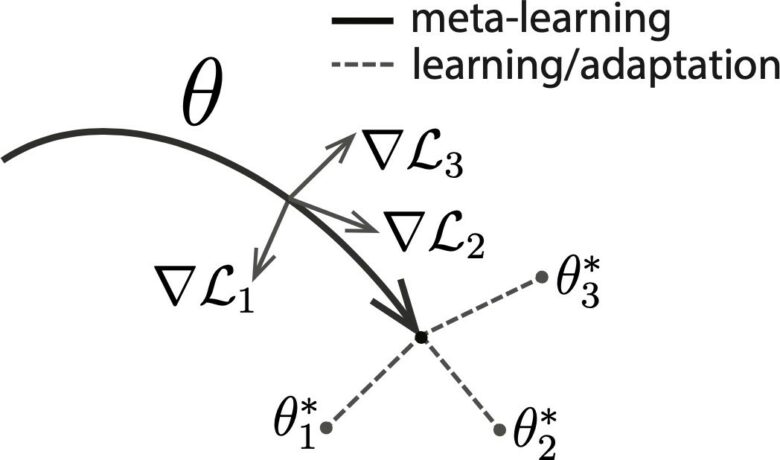Sunday, November 26, 2023
HomeAIWhat is meta-learning?

# What is meta-learning?

Therefore, in this blog, I will introduce introductory content about meta-learning, which is one of the methods of learning from a small amount of data.
Many of you may have heard the phrase, “Meta-learning is a method of learning how to learn.” I would appreciate it if you could have a good image.

## Contents

1. Positioning of meta-learning
2. Concrete method of meta-learning
3. in conclusion

## 1. Positioning of meta-learning

Meta-learning is a method that belongs to the framework of few-shot learning.
Few-shot learning is a problem setting in which different classes of data are given during training and testing. It is a method that aims to be able to make accurate predictions for test data belonging to a class that sometimes did not exist.

For example, suppose you wanted to classify lions and tigers in your test, but you didn’t collect many labeled images for lions and tigers, but you could collect labeled images for dogs and cats.
In this case, after training with images of dogs and cats, it is better to adapt the model to classify lions and tigers using only a few images of lions and tigers (usually around 3-5 images). It is called few-shot learning.

In practice, the Omniglot dataset is often used as a benchmark for evaluating the performance of few-shot learning and meta-learning.
This is a data set consisting of 1623 characters in 50 alphabets such as hiragana, katakana, Latin, and Greek.

This is divided into training data and test data, and after learning with the training data, only a few pieces of test data are used to adapt the model, and then the rest of the test data that was not used for adaptation We compare the performance of methods by measuring their accuracy.

In the case of this character recognition example, what is expected of meta-learning is to acquire the ability to successfully learn the common characteristics of all characters and quickly adapt to new characters, that is, to “learn how to learn.” It is to do meta-learning.

## 2. Concrete method of meta-learning

How do we “learn how to learn”?
Here, we introduce Model-Agnostic Meta-Learning (MAML) [Finn+, ICML’17], which is a representative method of meta-learning.
As an example, I will explain the case of using MAML in the situation where I am trying to classify the Omniglot data into 5 classes.
The procedure is as follows.

1. Divide the dataset into subsets called tasks.

At this time, each task is assigned 5 classes, and each task contains all the data of the assigned class.

1. Sample a set of tasks in the manner of mini-batch learning.
2. Perform steps 4 to 6 for each sampled task T i .
3. Data D for adaptation (5 sheets for each class, etc.) is sampled from task T i .
4. Using the sampled data D, the parameter Θ is updated by backpropagating the cross-entropy error in the same way as in normal supervised learning.

However, the parameter update here is for adaptation for each task, and the parameter of the parameter Θ adapted to the task T i is expressed as Θ’i , and the parameter Θ before update is not updated.

1. In order to evaluate whether the adaptation to the task is successful , data D’ i (15 sheets for each class, etc.) different from the previous D is sampled from the task T i .
2. For all the tasks included in the mini-batch, input the data D’i into the network with parameters Θ’i, calculate the cross-entropy error, and backpropagate the sum of the errors of all tasks to update the original parameters Θ.

Steps 2 to 7 are processed in one epoch, and are repeated many times until convergence.
Step 7 can be interpreted as evaluating “whether adaptation to each task is successful”.
Therefore, by repeating the above operation, we can understand that a small data set of 5 sheets for each class will become a network that can be well adapted to the task.

The figure below shows an image of what is done in MAML. By updating the network with the gradients (∇L 1 , ∇L 2 , ∇L 3
) for some tasks in MAML , we find the parameters of the network (Θ 1 , Θ 2 , Θ 3 are parameters adapted to different tasks).MAML is a proposal for a learning method and can be used for networks of any structure.
Hence the word model-agnostic in the name.

## 3. Conclusion

At Skill Up AI, we are currently offering a related course, ” Basic course for machine learning and data analysis that can be used in the field .”
In this course, you can learn the series of machine learning projects and the details of various algorithms through hands-on.

RELATED ARTICLES